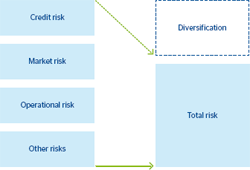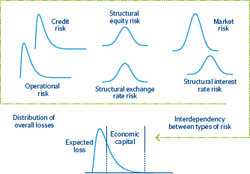# Integration of risks

The economic capital required to cover the Group’s losses is calculated by integrating the various risks managed by the Institution. The difference between this economic capital required and the sum of the individual capitals is known as the benefit of diversification, as the total capital needed is less than the sum of the individual capitals.

The BBVA Group’s risk integration model recognizes diversification among the various types of risks. The calculation process is divided into two stages. In the first stage, each of the risks is defined individually (credit, market, structural and operational), taking into account the special features in each case. In the second stage, they are added to a common measurement through a model that looks at the structure of dependency between risks.

In this framework, the diversification level of each of the risks depends, mainly, on the relative size of the risk against global risk, as well as the correlation among risks and the characteristics of individual loss distributions.

### Total risk and diversification### Integration of risksTools